# Performance Analysis of a Double-Sided PV Plant Oriented with Backtracking System

Performance Analysis of a Double-Sided PV Plant Oriented with Backtracking System

Francesco NicolettiMario A. Cucumo Vittorio Ferraro Dimitrios Kaliakatsos Jessica Settino

Department of Mechanical, Energy and Management Engineering (DIMEG), University of Calabria – Via P. Bucci - 87036, Rende (CS), Italy

Department of Computer, Modelling, Electronics and System Engineering (DIMES), University of Calabria – Via P. Bucci – 87036, Rende (CS), Italy

Corresponding Author Email:
francesco.nicoletti@unical.it
Page:
325-334
|
DOI:
https://doi.org/10.18280/mmep.070301
21 June 2020
|
Accepted:
18 August 2020
|
Published:
30 September 2020
| Citation

OPEN ACCESS

Abstract:

Among the new technologies for the exploitation of the solar source, double-sided photovoltaic panels represent a valid high efficiency solution. These are latest generation photovoltaic panels that allow you to capture solar radiation even from the back and to obtain a greater production of electricity compared to traditional single-sided panels. The performance of the panel is higher, with the consequent possibility of reducing the installation surface.

The paper defines a calculation model to analyse performances of a double-sided photovoltaic field, for both type of orientation N-S and E-W, in order to assess the effective increase in performance compared to a traditional system. Panels are equipped with a single-axis solar tracker, which changes their inclination so that they can have the best exposure to solar radiation.

The study also focuses on the optimal tracking methodology, in order to reduce the losses caused by shading. The applied technique is that of "backtracking". We have seen that backtracking is not useful for bifacial panels, but only for mono-facial.

In the work, a parametric analysis is also conducted to evaluate the increase in efficiency due to distance between rows of panels.

Keywords:

performance analysis, solar thermal generator, dish collector, flat mirrors

1. Introduction

Double-sided photovoltaic panels have existed for some time but only now, with the reduction of the price of solar cells, are they finding greater diffusion. These are photovoltaic panels that manage to absorb solar energy from both sides, increasing energy production compared to a standard photovoltaic module. This is, thus, a technology in spreading. Razongles et al.  in 2016, worried about the lack of standardization, wrote that investors are still reluctant to choose bifacial technologies. The reason was the absence of Standard Test Conditions for their measurement, and no available commercial simulator that can predict their energy production. They analysed, then, three different approaches for bifacial module performance measurements.

Different calculation models have been developed to evaluate the increase in performance due to the presence of a double layer of photovoltaics. This work will define a calculation model capable of estimating the producibility of a photovoltaic field for different tracking methods. In the literature there are several calculation models that have dealt with the prediction of performances. Chudinzow et al.  proposed a model to investigate the behaviour of bifacial PV power plants in more detail. They calculate the absorbed irradiation obtained from eight irradiance contributions for the front and rear of each cell string: direct and diffuse radiation arriving from sky and from ground. Their model was tested using a case study power plant placed in Chile.

A thermal steady-state model is defined by Zhang et al.  to evaluate the thermal performance of bifacial module and simulate it by ANSYS software. In particular they define a method to calculate the operating temperature of bifacial photovoltaic module.

Gu et al.  developed an optical-electrical-thermal model for the evaluation of bifacial PV performances. They obtained the global irradiances of the tilted front and rear surfaces through the optical model, the cell temperature through the thermal model, and the power output through the electrical model accordingly. Liang et al.  revised the pre-normative activity that has led to the draft standard IEC 60904-1-2. They showed the challenges in the selection of the non-reflective material behind the non-illuminated side, and their impact to the electrical characterization of bifacial modules. The model defined in this work allows to evaluate the unevenness of solar radiation incident on the ground due to the distances between the panels and the installation height. In fact, Important is also the non-uniformity of the rear irradiance . The double-sided modules can be used to obtain energy even where the application would not be advantageous with the classic coatings. Faturrochman et al.  used it for noise barriers. They developed a numerical model for predicting the power output for given weather conditions in the case of vertical installation.

In this work, instead, will also be analysed different types of handling and solar tracking in order to maximize the annual production of electricity. The cases of systems oriented in the N-S direction and in the E-W direction will be studied  with a tracking that minimizes the angle of incidence . The efficiency of the backtracking technique for double-sided photovoltaics will be verified. Backtracking is often used in single-sided photovoltaics to avoid the problem of shading generated at sunrise and sunset, when the rows of photovoltaic modules are arranged towards the horizon . From this point of view, servomechanisms orient the panels only in the central part of the day. In fact, at sunrise, sunset and during the night, the photovoltaic field is kept in a horizontal position with respect to the ground . Afterwards, when the shadows are contained, the panels begin to follow the solar trajectory. Many techniques have been developed to define the optimal backtracking strategy . Nascimento et al.  proposed an algorithm which can operate in any field slope avoiding the necessity of correcting the field slope where the solar tracker is placed. Lorenzo et al.  instead analysed the back-tracking strategy not only for single axis but also for two-axis tracking estimating corresponding energy gains. They showed that back-tracking is more useful for single horizontal axis than for the single vertical one, and on the other hand, that back-tracking is more efficient when applied in the primary axis of a two-axis tracker. In this work, however, the effectiveness of backtracking is assessed in the case of double-sided panels. In this case, it is investigated whether keeping the panels horizontal at sunrise and sunset could represent a deterioration in performance due to the little radiation collected from the rear. Furthermore, this system can also be combined with cooling systems [15, 16], which have not been analysed in this work bur can be the subject of future studies.

The defined calculation model also allows parametric evaluation of the action of some installation factors to define the optimal installation strategy.

2. Law of Motion Solar Tracking

The ability to rotate the photovoltaic panels by changing their tilt angle allows you to always work with a very low angle of incidence at any time of the day. This condition allows to exploit as much as possible the component of direct radiation from the sun. The procedure shown below refers to systems with a rotation axis along the North-South direction. To obtain the analogous information referred to the case of East-West orientation, it is sufficient to modify the apparent trajectory of the Sun by changing the solar azimuth by 90° . The position of the panels such as to minimize the angle of incidence can be deduced starting from Figure 1.

## 1.jpg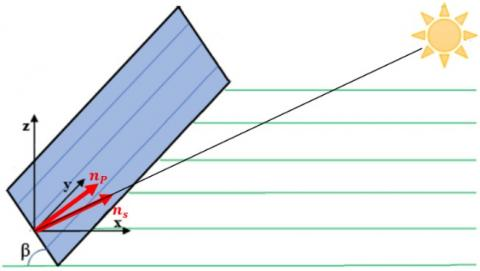Figure 1. Definition of the versors

The direction of the sun's rays can be schematized by means of the versor:

$n_{s}=\{\cos \alpha \sin \gamma ;-\cos \alpha \cos \gamma ; \sin \alpha\}$  (1)

where, $\alpha$ is the solar altitude and $\gamma$ the solar azimuth.

The versor that represents the normal to the surface of the panels (which can rotate around the North-South direction) expressed as a function of the tilt angle β is the following:

$n_{p}=\{\sin \beta ; 0 ; \cos \beta\}$ (2)

The cosine of the angle of incidence is given by the scalar product between the versors $n_{s}$ and $n_{p}$.

$\cos i=\cos \alpha \sin \gamma \sin \beta+\sin \alpha \cos \beta$  (3)

The goal is to identify the β angle that minimizes the angle of incidence. So, deriving Eq. (3).

$\frac{d \cos i}{d \beta}=\cos \alpha \sin \gamma \cos \beta-\sin \alpha \sin \beta=0$ (4)

From which it results:

$\beta=\arctan \left(\frac{\sin \gamma}{\tan \alpha}\right)$   (5)

For optimal use of photovoltaics, shading (partial or total) of the modules must be avoided. The shadow on the module is a cause of reduction of direct solar radiation incident on the panel. When designing a system, therefore, the possibility should be considered that the panels are sufficiently spaced apart to prevent them from causing even partial shading of the photovoltaic surface. With reference to Figure 2 it is possible to evaluate the shaded area due to the presence of adjacent panel, defined by the position of point B.

## 2.jpg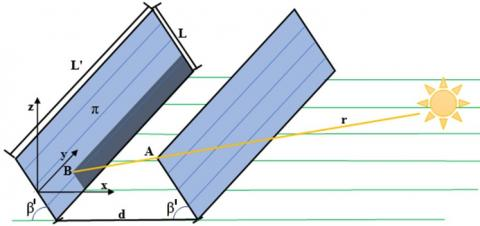A simplifying hypothesis deemed valid is to consider the shadow, rectangular in shape as shown in the Figure, although in reality it presents the shape of a rectangle trapezoid.

The straight-line r is defined as the parallel to the sun's rays and passing through point A, the vertex of the front panel. The projection of this point on the plane of the panel behind π, defines the projected shadow.

By fixing the reference system as in Figure 2, the parametric equation of the straight-line r is:

$\left\{\begin{array}{l}x=d-\frac{L}{2} \cos \beta^{\prime}+\cos \alpha \sin \gamma t \\ y=-\cos \alpha \sin \gamma t \\ z=\frac{L}{2} \sin \beta^{\prime}+\sin \alpha t\end{array}\right.$  (6)

where, L is the width of the panels and d is the distance between two adjacent ones, the angle $\beta^{\prime}$ is the angle of inclination of the panel which is equal to $\beta$ only if the inclination is that which minimizes the angle of incidence.

The equation of the π plane is:

$z=-\tan \beta^{\prime} x$  (7)

By solving the system of 4 equations given by Eq. (6) and (7), the intersection occurs for:

$t=-\frac{d \tan \beta^{\prime}}{\sin \alpha+\cos \alpha \sin \gamma \tan \beta^{\prime}}$  (8)

Substituting this parameter in the system of equations (6) we obtain the coordinates of point B $\left(x_{B}, y_{B}, z_{B}\right)$ .

Finally, to determine the area, if $\left(L^{\prime}-\left|y_{B}\right|\right)$ is less than 0 then there is no shadow ($A_{o m b}=0$), where $L^{\prime}$ is the length of the panels. If it is greater than 0, three cases are distinguished:

$A_{o m b}$$=\left\{\begin{array}{cc}0 & \text { if } \frac{L}{2}-\frac{x_{B}}{\cos \beta^{\prime}} \leq 0 \\ \left(\frac{L}{2}-\frac{x_{B}}{\cos \beta^{\prime}}\right)\left(L^{\prime}-\left|y_{B}\right|\right) & \text { if } 0<\frac{L}{2}-\frac{x_{B}}{\cos \beta^{\prime}} \leq L \\ L\left(L^{\prime}-\left|y_{B}\right|\right) & \text { if } \frac{L}{2}-\frac{x_{B}}{\cos \beta^{\prime}}>L\end{array}\right. (9) The shading factor is: f_{o m b}=1-\frac{A_{o m b}}{L^{\prime} \cdot L} (10) 4. Evaluation of the Ground Shading Based on the position of the sun and the panels, it is necessary to evaluate the solar irradiance reflected from the ground under the photovoltaic field. To recognize this rate it is necessary to determine the fraction of the ground placed under the photovoltaic field which is reached by direct radiation. The total area occupied by the photovoltaic field is as follows: A_{t o t}=L^{\prime} \cdot[N L+(N-1)(d-L)]=L^{\prime} \cdot L^{\prime \prime} (11) where, N is the number of rows of panels and L^{\prime \prime} is the width of the whole field. This area is partly fully illuminated and partly shaded with some illuminated strips. The area shaded by the panels placed at height H (Figure 3 and Figure 4) is determined by the of x* and y*, which are: x^{*}=\frac{\sin \gamma}{\tan \alpha} H (12) y^{*}=\frac{\cos \gamma}{\tan \alpha} H (13) The shaded area (including gaps) is: A_{o s c}=\left\{\begin{array}{lr}0 & \text { if }\left|x^{*}\right|>L^{\prime \prime} \text { or }\left|y^{*}\right|>L^{\prime} \\ \left(L^{\prime \prime}-\left|x^{*}\right|\right) \cdot\left(L^{\prime}-\left|y^{*}\right|\right) & \text { else }\end{array}\right. (14) ## 3.jpg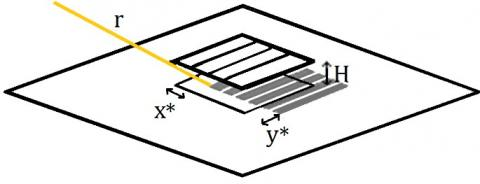Figure 3. Ground shading projected by panels ## 4.jpg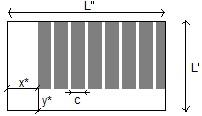Figure 4. Ground area shaded under solar field Within this area, however, the sun's rays penetrate through the spacing gaps between the various collectors. With reference to Figure 5, it is possible to determine the fraction f_{L}^{\prime} of A_{o s c} reached by direct radiation. In the Figure, the letter c represents the length of the shadow projected by a single panel, the angle 90^{\circ}-\beta represents the angle between the horizontal plane and the projection of the sun's rays on the vertical plane perpendicular to the direction of the rows of panels, the angle \beta^{\prime} is the angle of inclination of the panel, which only in the moments in which the backtracking takes place does not correspond to \beta. ## 5.jpgFigure 5. Ground area shaded under solar field The fraction of area A_{\text {osc}} irradiated by sunlight is: f_{L}^{\prime}=\frac{L^{\prime \prime}-N c}{L^{\prime \prime}} (15) Length c can be obtained using the sinus theorem applied to the triangle drawn under the first panel: \frac{c}{\sin \delta}=\frac{L}{\sin \left(90^{\circ}-\beta\right)} (16) where, \delta is an angle equal to \left[180^{\circ}-\beta^{\prime}-\left(90^{\circ}-\beta\right)\right]. After some mathematical steps: c=L \frac{\cos \left(\beta^{\prime}-\beta\right)}{\cos \beta} (17) Consequently: f_{L}^{\prime}=1-\frac{N L}{L^{\prime \prime}} \frac{\cos \left(\beta^{\prime}-\beta\right)}{\cos \beta} (18) Overall, the fraction of the total area under the photovoltaic field affected by direct irradiance is: f_{L}=\frac{\left(L^{\prime} L^{\prime \prime}-A_{o s c}\right)+f_{L}^{\prime} A_{o s c}}{L^{\prime} L^{\prime \prime}}=1-\frac{A_{o s c}}{L^{\prime} L^{\prime \prime}}\left(1-f_{L}^{\prime}\right) (19) 5. View Factors The basic hypothesis used to determine the view factors consists in considering the panels as infinitely long surfaces. They can therefore be assessed using the Hottel's crossed string rule. Figure 6 shows the schematic for identifying the crossed strings. The letters f and b indicate the front and rear surfaces of the photovoltaic panel, the letters t and s respectively represent the ground and the sky. ## 6.jpg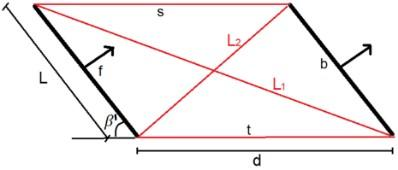Figure 6. Schematization for view factors identification View Factors F_{f-b} and F_{b-f} The crossed strings which connect that the surfaces f and b are L1 and L2: L_{1}=\sqrt{L^{2}+d^{2}+2 d L \cos \beta^{\prime}} (20) L_{2}=\sqrt{L^{2}+d^{2}-2 d L \cos \beta^{\prime}} (21) The not crossed strings are both equal to d. So applying the method, the factors of view F_{f-b} and F_{b-f} which for obvious reasons of symmetry are equal to each other, result: F_{f-b}=F_{b-f}=\frac{L_{1}+L_{2}-2 d}{2 L} View Factors F_{f-s} and F_{b-t} (22) The view factors F_{f-s} and F_{b-t} are also equal to each other by symmetry. In this case, the crossed strings have length equal to d and L, one uncrossed string has zero length and the other is equal to L_{2}. Consequently: F_{f-s}=F_{b-t}=\frac{L+d-L_{2}}{2 L} (23) View Factors F_{f-t} and F_{b-s} The crossed strings have length equal to d and L, one uncrossed string is null and the other is equal to L1. Consequently: F_{f-t}=F_{b-s}=\frac{L+d-L_{1}}{2 L} (24) 6. Incident Irradiance and PV Electric Power The incident irradiance on the photovoltaic surfaces is determined by the following expressions. Respectively G_{F} and G_{B} represent the irradiance incident on the front and on the back side of the panel. G_{F}=f_{\text {omb}} I_{\text {bo}} \frac{\cos i}{\sin \alpha}+I_{\text {do}} F_{f-s}+G_{B} \rho_{\mathrm{p}} F_{f-b}+$$+\left(I_{b o}+I_{d o}\right) \rho_{t} f_{L} F_{f-t}+I_{d o} \rho_{t}\left(1-f_{L}\right) F_{f-t}$  (25)

$G_{B}=I_{d o} F_{b-s}+G_{F} \rho_{\mathrm{p}} F_{b-f}+\left(I_{b o}+I_{d o}\right) \rho_{t} f_{L} F_{b-t}$$+I_{d o} \rho_{t}\left(1-f_{L}\right) F_{b-t}$  (26)

where, $I_{b o}$ and $I_{d o}$ are direct and diffuse solar irradiance on the horizontal plane; $\rho_{\mathrm{p}}$ is the reflectivity of the photovoltaic panel, $\rho_{\mathrm{t}}$ is the albedo.

On the front surface the terms of the summation in Eq. (25) indicate:

• direct radiation, taking into account the shading and the inclination factor;
• diffuse radiation from the atmosphere;
• total radiation reflected from the rear part of the adjacent panel;
• radiation reflected from the ground directly affected by sunlight;
• diffuse radiation reflected from the ground not affected by sunlight.

On the back side of panel the components are the same except for direct radiation from the sun. It is a system of equations in unknowns $G_{F}$ and $G_{B}$ easily solvable. The electric power per square meter of panel $P_{e}$ is obtained by considering the yield estimated with the simplified formula given by Evans .

$\eta=\eta_{R}\left[1-\beta^{*}\left(T_{c}-T_{R}\right)\right]$  (27)

where, $\eta_{R}$ and $T_{R}$ are the reference efficiency and the reference temperature of the photovoltaic panel, $\beta^{*}$ is a constant that depend on the material of which the modules are made (for silicon solar cells $\beta^{*}=0,0045 \frac{1}{^\circ C}$), G is the irradiance incident on the surface, $T_{C}$ is the cell temperature which, for simplicity, has been set equal to environment temperature. To precisely define its temperature, models that descend to a greater level of detail would be needed . The electrical power per m2 is:

$P_{e}=\eta G_{t o t}$  (28)

where, $G_{t o t}$ is the sum of the two contributions of incident solar irradiance.

7. Analysis of Results

The analysis is conducted by studying different orientations and methods of solar tracking and assessing the annual producibility of the system. The temperature and direct and diffused solar radiation data are taken from the PVGIS database  relating to the city of Cosenza (Italy) with a latitude of 39.3° N. The simulations are conducted in a stationary way with one-minute time intervals. Since the meteorological data provided by the PVGIS are hourly, the minute-by-minute values are obtained by linear interpolation.

The panel positioning technique analyzed in the simulations is summarized in these four case studies:

1. Panels arranged with the rotation axis in the North-South direction and moved according to the law defined by the Eq. (5);
2. Panels arranged horizontally not moved;
3. Panels arranged with the rotation axis in the East-West direction and moved according to the law defined by the Eq. (5);
4. Panels arranged with the rotation axis in the East-West direction and inclined by 30° in the South direction not moved.

The electrical and geometric data and reflectivity used for the simulations are shown in the Table 1.

Table 1. Geometric and electrical data

 Geometric data Electrical data L 1 m $\eta_{R}$ 0.152 d 1,6 L $T_{R}$ 25 H L Reflectivity L' 8 m $\rho_{P}$ 0.1 N 8 $\rho_{t}$ 0.2

Figures 7, 8, 9, 10 show the daily trends of the incident irradiance on the front surface, on the rear surface and total irradiance $G_{t o t}$ for the winter solstice. The incidence radiation on the rear is significant in both cases where movement is present. Furthermore, for the E-W orientation, the incident radiative contribution on the rear surface is high at noon, this does not happen for the N-S orientation.

## 7.jpgFigure 7. Incident irradiance for N-S field with solar tracking. Winter solstice

## 8.jpg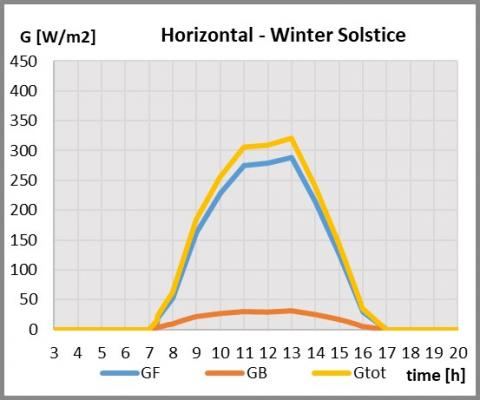Figure 8. Incident irradiance for horizontal field. Winter solstice

## 9.jpgFigure 9. Incident irradiance for E-W field with solar tracking. Winter solstice

## 10.jpgFigure 10. Incident irradiance for E-W field with tilt angle of 30° south. Winter solstice

## 11.jpgFigure 11. Incident irradiance for N-S field with solar tracking. Summer solstice

It is necessary to compare the total incident radiation among all types of system. Figure 15 shows the $G_{t o t}$ trends with reference to the winter replacement. It is interesting to note that the E-W system inclined by 30° in South is the one that receives the maximum radiation. This is due to less shading, which make the direct incident component high for 30° south plant (at 12 o'clock, in fact, $f_{\text {omb}}$ is 87.6%, while in the case of E-W orientation with tracking $f_{\text {omb}}$ is 74.4%). The system with N-S orientation with tracking, on the other hand, has less radiation because, in winter, the angle of incidence, especially in the central hours of the day, stands at higher values. Figure 16 compares the same quantities in the case of a summer solstice. The figure shows that the systems with tracking perform better than in cases without movement, this is due to the lesser shading that occurs in the summer.

Therefore, during the winter solstice, representative of the winter months, the plant oriented in the N-S direction with solar tracking does not behave in an excellent way. On the contrary, however, this orientation is convenient in the summer solstice.

To evaluate which tracking methodology is the best, it is necessary to estimate the total annual electrical producibility. Since the incident radiation depends a lot on the season, the results obtained for electricity produced per unit of square meter E [kWh/m2] for each month of the year, for each system, is shown in Table 2. In order to assess the influence of the double-sided system, the results of the classic single-sided panels are also present in the same table.

## 12.jpgFigure 12. Incident irradiance for horizontal field. Summer solstice

## 13.jpgFigure 13. Incident irradiance for E-W field with solar tracking. Summer solstice

## 14.jpg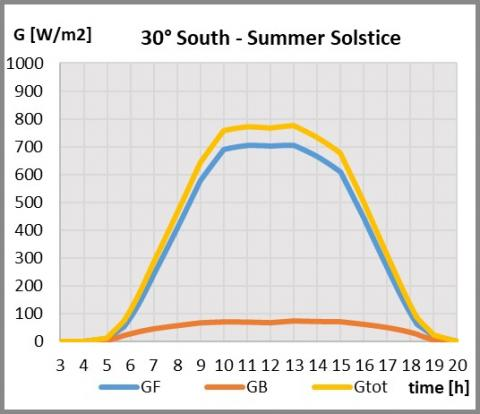Figure 14. Incident irradiance for E-W field with tilt angle of 30° south. Summer solstice

## 15.jpg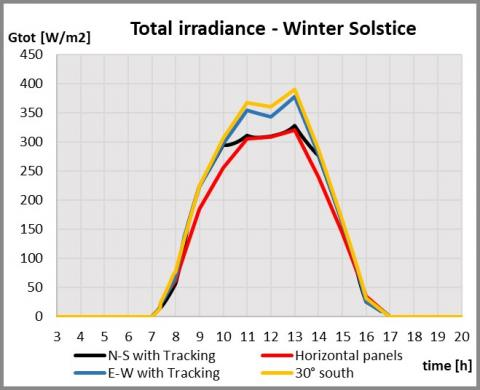Figure 15. Total incident irradiance. Winter solstice

## 16.jpgFigure 16. Total incident irradiance. Summer solstice

Table 2. Electric energy per m2 for different strategies

 E [kWh/m2] Jan Feb Mar Apr May Jun Jul Aug Sep Oct Nov Dec Tot Mono-facial PV N-S with Tracking 9.4 10.0 22.2 25.8 34.6 36.1 42.3 33.0 21.2 19.6 11.7 12.1 278 Horizontal panels 8.9 9.9 20.9 24.5 32.0 32.9 37.8 30.1 20.2 18.0 10.8 11.2 257 E-W with Tracking 10.1 10.9 23.2 25.1 32.7 33.3 38.6 31.1 21.4 21.9 12.9 13.4 275 30° South 11.1 11.7 23.7 24.6 30.0 29.4 34.9 29.7 21.7 22.2 13.9 14.3 267 Bifacial PV N-S with Tracking 10.7 11.3 24.6 28.5 37.7 39.3 45.7 36.0 23.5 21.8 13.2 13.6 306 Horizontal panels 10.0 10.9 23.0 26.8 34.9 35.8 41.0 32.9 22.2 20.0 12.1 12.5 282 E-W with Tracking 11.6 12.3 25.4 27.5 35.6 36.4 42.0 33.9 23.5 24.0 14.5 15.0 302 30° South 11.9 12.5 25.5 27.1 33.4 33.0 38.8 32.7 23.6 23.7 14.9 15.3 293

For a better reading of the table, the monthly production is shown in graphic form in Figures 17 and 18 respectively for mono-facial PV and bifacial PV. From the two figures it can be observed that for each type of movement, the electrical production of the double-sided PV has improved in proportion to the single-sided case. In practice, the presence of photovoltaics on the rear part does not favor one system over another. All the bars of Figure 18 are higher than Figure 17, in approximately the same proportion.

## 17.jpgFigure 17. Electric energy per m2 for mono-facial field

## 18.jpgFigure 18. Electric energy per m2 for Bifacial field

Important are the data relating to the annual electrical production visible in Table 2, which allow to evaluate the actual increase in performance due to the presence of the bifacial PV (bifacial gain) in the case examined of d=1.6 L:

• For N-S with Tracking the production is 306 kWh/m2 (10.1% more than the single-sided PV).
• for horizontal panels the production is 282 kWh/m2 (9.7% more than the single-sided PV);
• for E-W with Tracking the production is 302 kWh/m2 (9.8% more than the single-sided PV);
• for 30° South panels the production is 293 kWh/m2 (9.7% more than the single-sided PV)

In percentage terms, the bifacial gain is about the same for each type studied.

It is important to underline that the data obtained are strictly linked to the installation distance between photovoltaic modules. The results shown above refer to d = 1.6 L. Figure 19 shows how the electricity produced per m2 varies according to the spacing. The influence of the spacing is marked for values of d/L lower than 2.5. In particular, for systems with tracking, the production is approximately the same for d/L<1.7, for higher values the production obtained with the N-S orientation is greater. The figure also shows that, if the panels are not spaced, it is convenient to keep them always in a horizontal position, despite the incident angle is low and radiation on the rear part is not high.

## 19.jpgFigure 19. Annual electric energy per m2 as a function of spacing

The bifacial gain also depends on the mutual distance between two panels. Figure 20 shows the convenience of using the double-sided modules for the four systems analyzed. In particular, in cases where the parameter d/L is between 1.6 and 1.9 the resulting gain is approximately the same for all systems, as also seen previously for the particular case of 1.6.

## 20.jpgFigure 20. Bifacial gain as a function of spacing

For distances of less than 1.6, the N-S with tracking system allows you to better exploit the rear part, while with the horizontal panels, the ground is too dark to provide a consistent contribution of radiation. While, for d/L values higher than 1.9, the bifacial gain is higher for horizontal panels than the other systems, however, the energy obtained as shown in Figure 19 is still lower than the others. The fields oriented in the E-W direction with and without tracking have an intermediate behavior and the bifacial gain is approximately equal to each other.

Finally, with reference to the plant oriented in the N-S direction, which represents the solution that guarantees greater producibility at an annual level, an additional strategy for positioning the panels over time has been analyzed. The tracking studied so far was such that the panels were always positioned in such a way as to minimize the incidence angle. This situation is not such as to maximize the incident irradiance at any time of the day, due to the shading that occurs when the solar height is low. In these conditions, it is also evident from the results previously shown that keeping the panels in a horizontal position could be more convenient. The backtracking technique is a movement strategy according to which the panels are kept in a horizontal position until the solar altitude reaches an adequate $\alpha_{l i m}$ value, after which the movement continues following the law of Eq. (5) of minimum incidence. In mathematical terms the law of motion can be summarized as follows:

$\beta^{\prime}=\left\{\begin{array}{ll}0 & \text { if } \quad \alpha<\alpha_{l i m} \\ \beta & \text { if } \quad \alpha \geq \alpha_{\text {lim }}\end{array}\right.$  (29)

The aim is to identify what the limit condition $\alpha_{l i m}$ is that maximizes electricity production. A parametric analysis is therefore conducted to determine the optimal value and estimate the increase in producibility. In the case of panels slightly spaced from each other with d/L = 1.3, The results obtained are shown in Figure 21. The figure shows the backtracking gain obtained for both single-sided and double-sided panels as a function of $\alpha_{l i m}$. In the event that the panels are single-sided, $\alpha_{l i m}$ optimal results of about 24° with a gain of about 2.2%. Note that 24° represents a very high value, for the latitude considered it means that in winter the panels are almost always arranged horizontally. In the case of double-sided panels, however, the curve turns out to be very different. An optimum value is obtained at 13° and the increase in producibility is 0.75%.

Again, the results depend a lot on the d/L ratio. Figure 22 shows the case in which it is equal to 1.6 L. The optimal values of $\alpha_{l i m}$ are different compared to the previous case and the gain is also much lower: in fact, the shading is not relevant. In the case of single-sided panels the optimal limit angle is 12° with a gain of 0.65%, while in the case of double-sided systems the gain is only 0.4% with an $\alpha_{l i m}$ of 10°.

## 21.jpg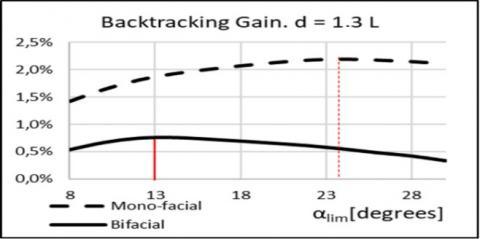Figure 21. Backtracking gain as a function solar altitude limit with d/L=1.3

## 22.jpg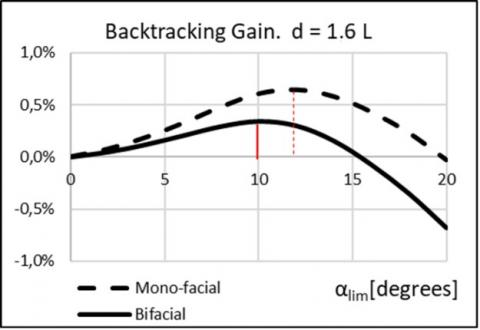Figure 22. Backtracking gain as a function solar altitude limit with d/L=1.6

8. Conclusions

The bifacial photovoltaic modules represent a low-cost solution that allows you to double the installed system power for the same occupied area. This particularity represents an interesting solution to maximize electricity production in the event that a limited area is available. The work proposed a methodology for the calculation of the electrical power produced instantly based on the characteristics of the panel and the inclination adopted. A determining factor to exploit the photovoltaic rear part is the distance between adjacent collectors. In fact, it affects both the mutual shading and the view factors between the rear part and surfaces with different radiance. In the proposed calculation method, it is necessary to estimate the amount of shading also on the ground, which has a significant impact on the incident radiation on the rear. Various possible orientations of the panels rotated with different laws of motion were analyzed, in order to investigate which was the best one with reference to a location at 39.3° latitude. The most convenient option is represented by panels oriented north-south with monoaxial tracking. In particular, this solution is very performing in the summer period. The presence of photovoltaic in the rear part, in this case, allows to increase the electricity production, at an annual level, by about 10.1% with a spacing equal to 1.6 times the width of the panels. However, the bifacial gain increases, with increasing distance.

In addition, the benefits of backtracking have been studied. Through this strategy it is possible to cancel the shading at sunrise and sunset by keeping the panels in a horizontal position. The treatment was carried out for single-sided and double-sided modules with N-s orientation. it has been found that for the double-sided modules the gain is not particularly high (below 1%). As regards single-sided systems, the convenience is also 2% where the panels are not far apart. However, since the plant already has the tracking system, the adoption of this technique is at no cost and it is useful to use backtracking also to reduce the mechanical stresses caused by the wind.

Acknowledgement

This research is funded by Regione Calabria (PAC CALABRIA 2014-2020 - Asse Prioritario 12, Azione B) 10.5.12 - CUP H28D19000040006).

Nomenclature
 $A_{o m b}$ shaded photovoltaic area, m2 $A_{\text {osc }}$ shaded area under field, gaps included, m2 $A_{t o t}$ total area occupied by solar field, m2 c width of panel projected to the ground by sun, m d distance between two rows of panels, m E Electric energy, kWh $f_{L}$ fraction of $A_{t o t}$  reached by direct irradiance $f_{L}^{\prime}$ fraction of $A_{o s c}$  reached by direct irradiance $f_{\text {omb}}$ fraction of pv area reached by direct irradiance Fi-j View factor between i and j surfaces GF incident irradiance on the front surface, W.m-2 GB incident irradiance on the rear surface, W.m-2 Gtot total incident irradiance (GF + GB), W.m-2 H height of the panels, m i incidence angle (rad) Ib,o direct solar irradiation on horizontal plane, W.m-2 Id,o diffuse solar irradiation on horizontal plane, W.m-2 L width of the panels, m L’ length of row of panels, m L’’ width of the whole field, m L1 crossed string 1, m L2 crossed string 2, m N number of rows of panels np versor of the normal of the panel ns versor of sun position Pe Electric power, W r straight line of sunlight T temperature, K x, y, z spatial coordinates, m x*, y* spatial coordinates of vertex of shaded area, m Greek symbols α solar altitude, rad β tilt angle that minimize the incidence angle, rad β’ tilt angle, rad β* temperature coefficient, K-1 γ solar azimuth, rad δ construction angle, rad η pv yield ηR reference pv yield π panel plane ρP photovoltaic reflectivity ρt albedo Subscripts b back side of panel B vertex of photovoltaic panel f front side of panel R reference s sky t ground P panel
References

 Razongles, G., Sicot, L., Joanny, M., Gerritsen, E., Lefillastre, P., Schroder, S., Lay, P. (2016). Bifacial photovoltaic modules: measurement challenges. Energy Procedia, 92: 188-198. https://doi.org/10.1016/j.egypro.2016.07.056

 Chudinzow, D., Haas, J., Díaz-Ferrán, G., Moreno-Leiva, S., Eltrop, L. (2019). Simulating the energy yield of a bifacial photovoltaic power plant. Solar Energy, 183: 812-822. https://doi.org/10.1016/j.solener.2019.03.071

 Zhang, Z., Wu, M., Lu, Y., Xu, C., Wang, L., Hu, Y., Zhang, F. (2020). The mathematical and experimental analysis on the steady-state operating temperature of bifacial photovoltaic modules. Renewable Energy, 155: 658-668. https://doi.org/10.1016/j.renene.2020.03.121

 Gu, W., Ma, T., Li, M., Shen, L., Zhang, Y. (2020). A coupled optical-electrical-thermal model of the bifacial photovoltaic module. Applied Energy, 258. https://doi.org/10.1016/j.apenergy.2019.114075

 Liang, T.S., Poh, D., Pravettoni, M. (2018). Challenges in the pre-normative characterization of bifacial photovoltaic modules. Energy Procedia, 150: 66-73. https://doi.org/10.1016/j.egypro.2018.09.006

 Ledesma, J.R., Almeida, R.H., Martinez-Moreno, F., Rossa, C., Martín-Rueda, J., Narvarte, L., Lorenzo, E. (2020). A simulation model of the irradiation and energy yield of large bifacial photovoltaic plants. Solar Energy, 206: 522-538. https://doi.org/10.1016/j.solener.2020.05.108

 Faturrochman, G.J., De Jong, M.M., Santbergen, R., Folkerts, W., Zeman, M., Smets, A.H.M. (2018). Maximizing annual yield of bifacial photovoltaic noise barriers. Solar Energy, 162: 300-305. https://doi.org/10.1016/j.solener.2018.01.001

 Cucumo, M., Ferraro, V., Kaliakatsos, D., Mele, M., Nicoletti, F. (2017). Law of motion of reflectors for a linear Fresnel plant. International. Journal of Heat and Technology, 35(1): S78-S86. https://doi.org/10.18280/ijht.35Sp0111

 Bruno, R., Bevilacqua, P., Longo, L., Arcuri, N. (2015). Small size single-axis PV trackers: Control strategies and system layout for energy optimization. Energy Procedia, 82: 737-743. https://doi.org/10.1016/j.egypro.2015.11.802

 Lamigueiro, O.P. (2012). solaR: Solar radiation and photovoltaic systems with R. Journal of Statistical Software, 50(9): 1-32. https://doi.org/10.18637/jss.v050.i09

 Lorenzo, E., Pérez, M., Ezpeleta, A., Acedo, J. (2002). Design of tracking photovoltaic systems with a single vertical axis. Progress in Photovoltaics: Research and Applications, 10(8): 533-543. https://doi.org/10.1002/pip.442

 Esram, T., Chapman, P.L. (2007). Comparison of photovoltaic array maximum power point tracking techniques. IEEE Transactions on Energy Conversion, 22(2): 439-449. https://doi.org/10.1109/TEC.2006.874230

 Albuquerque, D., Nascimento, B., Lima, M., Sousa, P. (2015). Backtracking algorithm for single-axis solar trackers installed in a sloping field. International Journal of Engineering Research and Applications, 5(12): 100-103.

 Lorenzo, E., Narvarte, L., Muñoz, J. (2011). Tracking and back-tracking. Progress in Photovoltaics: Research and Applications, 19(6): 747-753. https://doi.org/10.1002/pip.1085

 Bevilacqua, P., Bruno, R., Arcuri, N. (2020). Comparing the performances of different cooling strategies to increase photovoltaic modules electric in different meteorological conditions. Energy, 195: 116950. https://doi.org/10.1016/j.energy.2020.116950

 Bevilacqua, P., Morabito, A., Bruno, R., Ferraro, V., Arcuri, N. (2020). Seasonal performances of photovoltaic cooling systems in different weather conditions. J. Clean. Prod, 272: 122459. https://doi.org/10.1016/j.jclepro.2020.122459

 Cucumo, M.A., Ferraro, V., Kaliakatsos, D., Nicoletti, F. (2019). Study of kinematic system for solar tracking of a linear Fresnel plant to reduce end losses. European Journal of Electrical Engineering, 21(5): 393-400. https://doi.org/10.18280/ejee.210501

 Evans, D.L (1981). Simplified method for predicting photovoltaic array output. Solar Energy, 27: 555-560. https://doi.org/10.1016/0038-092X(81)90051-7

 Bevilacqua, P., Perrella, S., Bruno, R., Arcuri N. (2020). An accurate thermal model for the PV electric generation prediction: Long-term validation in different climatic conditions. Renewable Energy, 163: 1092-1112. https://doi.org/10.1016/j.renene.2020.07.115

 Huld, T., Müller, R., Gambardella, A. (2012). A new solar radiation database for estimating PV performance in Europe and Africa. Solar Energy, 86: 1803-1815. https://doi.org/10.1016/j.solener.2012.03.006